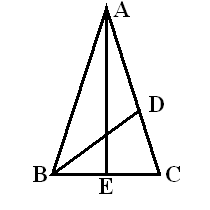## Multiples of 18 Degrees

Trigonometry texts always include material early in the course on finding the exact values of trig functions of the angles $0^\circ, 30^\circ, 45^\circ, 60^\circ$, and $90^\circ$. It is also true that by a similar argument, exact values of trig functions of the angles $18^\circ, 36^\circ, 54^\circ$, and $72^\circ$ may also be found. These angles are equivalent to unit circle arc lengths that are multiples of $\dfrac{\pi}{10}$.

### Trig Values of Multiples of $\dfrac{\pi}{10}$

 $\theta$ $\sin\theta$ $\cos\theta$ $\tan\theta$ $\cot\theta$ $\sec\theta$ $\csc\theta$ $\dfrac{\pi}{10}$ $\dfrac{\sqrt{5}-1}{4}$ $\dfrac{\sqrt{10+2\sqrt{5}}}{4}$ $\dfrac{\sqrt{25-10\sqrt{5}}}{5}$ $\sqrt{5+2\sqrt{5}}$ $\dfrac{\sqrt{50-10\sqrt{5}}}{5}$ $\sqrt{5}+1$ $\dfrac{\pi}{5}$ $\dfrac{\sqrt{10-2\sqrt{5}}}{4}$ $\dfrac{1+\sqrt{5}}{4}$ $\sqrt{5-2\sqrt{5}}$ $\dfrac{\sqrt{25+10\sqrt{5}}}{5}$ $\sqrt{5}-1$ $\dfrac{\sqrt{50+10\sqrt{5}}}{5}$ $\dfrac{3\pi}{10}$ $\dfrac{1+\sqrt{5}}{4}$ $\dfrac{\sqrt{10-2\sqrt{5}}}{4}$ $\dfrac{\sqrt{25+10\sqrt{5}}}{5}$ $\sqrt{5-2\sqrt{5}}$ $\dfrac{\sqrt{50+10\sqrt{5}}}{5}$ $\sqrt{5}-1$ $\dfrac{2\pi}{5}$ $\dfrac{\sqrt{10+2\sqrt{5}}}{4}$ $\dfrac{\sqrt{5}-1}{4}$ $\sqrt{5+2\sqrt{5}}$ $\dfrac{\sqrt{25-10\sqrt{5}}}{5}$ $\sqrt{5}+1$ $\dfrac{\sqrt{50-10\sqrt{5}}}{5}$

Proof: Let $A$ be the point   $(1,0)$   on the unit circle, and choose points $B$, $C$, $D$, and $E$ on the unit circle counterclockwise from $A$ so that arc lengths $AB$, $BC$, $CD$, $DE$, and $EA$ are each $\dfrac{2\pi}{5}$. Let   $(x,y)$   be the coordinates of point $B$, and let   $(v,w)$   be the coordinates of point $C$. Then, by symmetry, the coordinates of point $D$ are   $(v,-w)$,   and the coordinates of point $E$ are   $(x,-y)$.   Since all of these points are on the unit circle, the equations   $x^2+y^2=1$   and   $v^2+w^2=1$   are both true.Since the line segments $AB$ and $BC$ are equal, we apply the distance formula to obtain the equation   $(x-1)^2+y^2=(x-v)^2+(y-w)^2$,   which simplifies to   $yw=x-xv$.   Similarly, the equality of the line segments $AC$ and $BD$ will produce an equation which simplifies to   $yw=xv-v$.   Equating these results gives   $v=\dfrac{x}{2x-1}$.   The equality of the line segments $AB$ and $CD$ will produce an equation which simplifies to   $4w^2=2-2x$.   Using   $v^2+w^2=1$   to substitute for the variable $w$, then using   $v=\dfrac{x}{2x-1}$   to substitute for the variable $v$, then removing fractions, we obtain the polynomial equation   $8x^3-4x^2-6x+2=0$.   Since 1 is a solution of this equation, the polynomial factors into a linear and a quadratic expression. Since point $B$ is in the first quadrant, the value of $x$ must be positive, and the quadratic formula produces   $x=\dfrac{-1+\sqrt{5}}{4}$.   The values of the variables $y$, $v$, and $w$ then can be produced by substitution.

The values of the sine and cosine functions are then related to the variables through symmetry arguments as follows:

The values of the other four trigonometric functions can be found with the ratio and reciprocal identities.♦

Alternate Proof: Suppose triangle $ABC$ is isosceles, with congruent sides $AB$ and $AC$ each of length 1, and the measure of angle $BAC$ is $36^\circ$. Then angles $ABC$ and $ACB$ are also congruent, and since the sum of the angles of a triangle is $180^\circ$, the measure of each of these two angles is $72^\circ$.Now bisect angle $ABC$, and let point $D$ be the intersection of side $AC$ with the bisector of angle $ABC$. Now angle $ABD$ is $36^\circ$, so triangle $ABD$ is isosceles, with congruent sides $AD$ and $BD$. Also, since angle $DBC$ is $36^\circ$ and angle $BCD$ is $72^\circ$, we find that angle $BDC$ must be $72^\circ$, therefore triangle $BDC$ is isosceles with congruent sides $BD$ and $BC$. Note that triangles $ABC$ and $BCD$ are similar triangles, by AAA Similarity.

Let $x$ be the length of side $BC$ (which is also the length of $BD$ and of $AD$). Then by similar triangles, we obtain the proportion   $\dfrac{AB}{BC}=\dfrac{BC}{CD}$,   which is equivalent to   $\dfrac{1}{x}=\dfrac{x}{1-x}$.   Solving this equation by cross-multiplying, using the quadratic formula and discarding the negative solution, we obtain   $x=\dfrac{\sqrt{5}-1}{2}$.

Now bisect angle $BAC$, and let point $E$ be the intersection of side $BC$ with the bisector of angle $BAC$. Since triangle $ABC$ is isosceles, triangles $ABE$ and $ACE$ are congruent, and by corresponding parts $CE$ is half the measure of $BC$, and angle $AEC$ is a right angle.

Triangle $ACE$ is now observed to be a right triangle whose acute angles measure $18^\circ$ and $72^\circ$, and with sides of lengths   $AC = 1$   and   $CE=\dfrac{\sqrt{5}-1}{4}$.   By the definitions of sine and cosine, we have   $\sin 18^\circ =\cos 72^\circ =\dfrac{\sqrt{5}-1}{4}$.   All other results can be obtained through the use of sum, difference, and double angle formulas, and the Pythagorean identity.♦